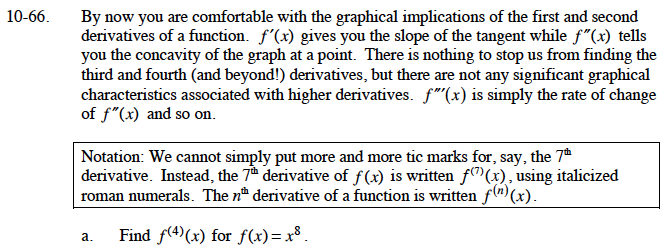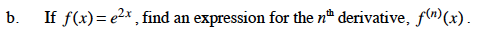### Home > CALC > Chapter 10 > Lesson 10.1.7 > Problem10-66

10-66.
1. By now you are comfortable with the graphical implications of the first and second derivatives of a function. f ′(x) gives you the slope of the tangent while f ″(x) tells you the concavity of the graph at a point. There is nothing to stop us from finding the third and fourth (and beyond!) derivatives, but there are not any significant graphical characteristics associated with higher derivatives. f ′″(x) is simply the rate of change of f ″(x) and so on.

Notation: We cannot simply put more and more tic marks for, say, the 7th derivative. Instead, the 7th derivative of f(x) is written f (7) (x), using italicized roman numerals. The nth derivative of a function is written f (n) (x). Homework Help ✎

1. Find f (4) (x) for f(x) = x8.

2. If f(x) = e2x , find an expression for the nth derivative, f (n) (x).Take the derivative four times.Take a few derivatives of this function and look for patterns.